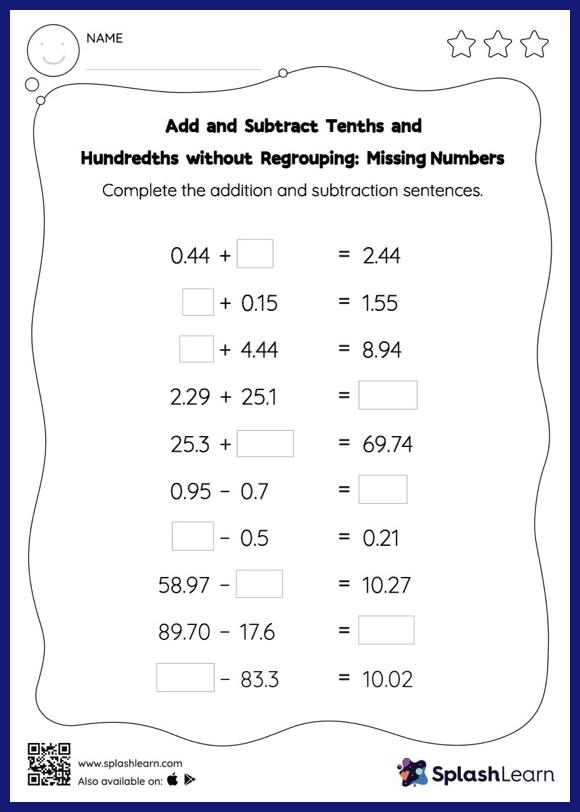# Add and Subtract Tenths and Hundredths without Regrouping: Missing Numbers Worksheet

Home > Add and Subtract Tenths and Hundredths without Regrouping: Missing NumbersAdd and subtract tenths and hundredths without regrouping worksheet helps students develop proficiency with addition and subtraction. While adding and subtracting decimals, students use the relationship between addition and subtraction to find the missing number. They do not need to regroup numbers in add and subtract tenths and hundredths without regrouping worksheet. In each problem, the numbers are laid out in the horizontal format. Students should try to use different strategies involving composing and decomposing numbers to solve these problems. This will help them develop flexibility and fluency.